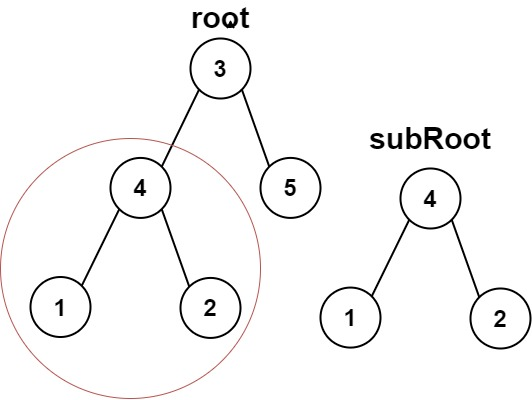# Leetcode 572 - Subtree of another tree

Note:

• Need a helper isSameTree
• Recursively to check if isSame(node, subRoot) is true.

Given the roots of two binary trees root and subRoot, return true if there is a subtree of root with the same structure and node values of subRoot and false otherwise.

A subtree of a binary tree tree is a tree that consists of a node in tree and all of this node’s descendants. The tree tree could also be considered as a subtree of itself.

Example